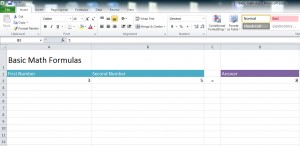# Basic Math in Excel

6
JanOne of Excel’s most fundamental utilizations is its ability to formulate and solve basic math for the user. Basic math in Excel is done through easy formula creation that can help with reports, charts, budgeting, and more.

To begin creating basic math formulas, choose two numbers. For the example, we will use “2” and “5” for an addition problem.

Place the number 2 in the cell “A2” and the number 5 in the cell “B2”. Once this is done, there are two ways to solve the equation through Excel functions:

• You can use the Excel AutoSum function.
• Write the formula out for yourself.

## How to use the AutoSum Function in Excel

Click the tab labeled “Formulas” at the top of the Excel Ribbon. The first option to the top left is what you need:

Next, choose the down arrow next to “AutoSum” and click on “Sum”. In the top input bar for cell formulas, it will display “=SUM()”. Select the empty cell where you want the answer to appear, such as “C2”.

Fill in the parenthesis with the formula A2:B2 so it looks like this:

Once you have created that, the answer “7” will appear in the cell you selected, “C2”. with this formula, you can change the number in “A2” to something like “3”, the cell C2 will automatically update to “8”.

## Writing Excel Formulas Yourself

If you choose to write basic formulas yourself, you can use more than just addition. Subtraction, division, and multiplication are all easy to write into Excel.

Using the example file, enter a number into A2 and another in B2. In the D2 cell under “Answers” is where the formula will appear when you’re finished.

In the formula bar, enter for addition:

=a2+b2

For subtraction:

=a2-b2

Multiplication is through an asterisk:

= a2*b2

Division is represented with a slash:

= a2/b2

Once you have entered a formula, the answer will appear in the D2 cell.